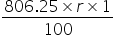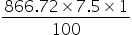# CAT-2021-QA-Slot-1/Free CAT Question papers

10).

Anil invests some money at a fixed rate of interest, compounded annually. If the interests accrued during the second and third year are ₹ 806.25 and ₹ 866.72, respectively, the interest accrued, in INR, during the fourth year is nearest to

1. Rs 931.72

2. Rs 926.84

3. Rs 929.48

4. Rs 934.65

### Explanation

Let the rate of interest is r % per annum

The interest accrued during the second year = ₹ 806.25

The interest accrued during the third year = ₹ 866.72

The extra interest in the third year = 866.72 – 806.25 = ₹ 60.47 is the interest for one year on

the second year interest

60.47 == >  r = 7.5%

Interest for the fourth year = Interest for third year + interest for one year on third year interest

= 866.72 += 866.72 + 65 =  ₹  931.72 approximately

Ans (1)